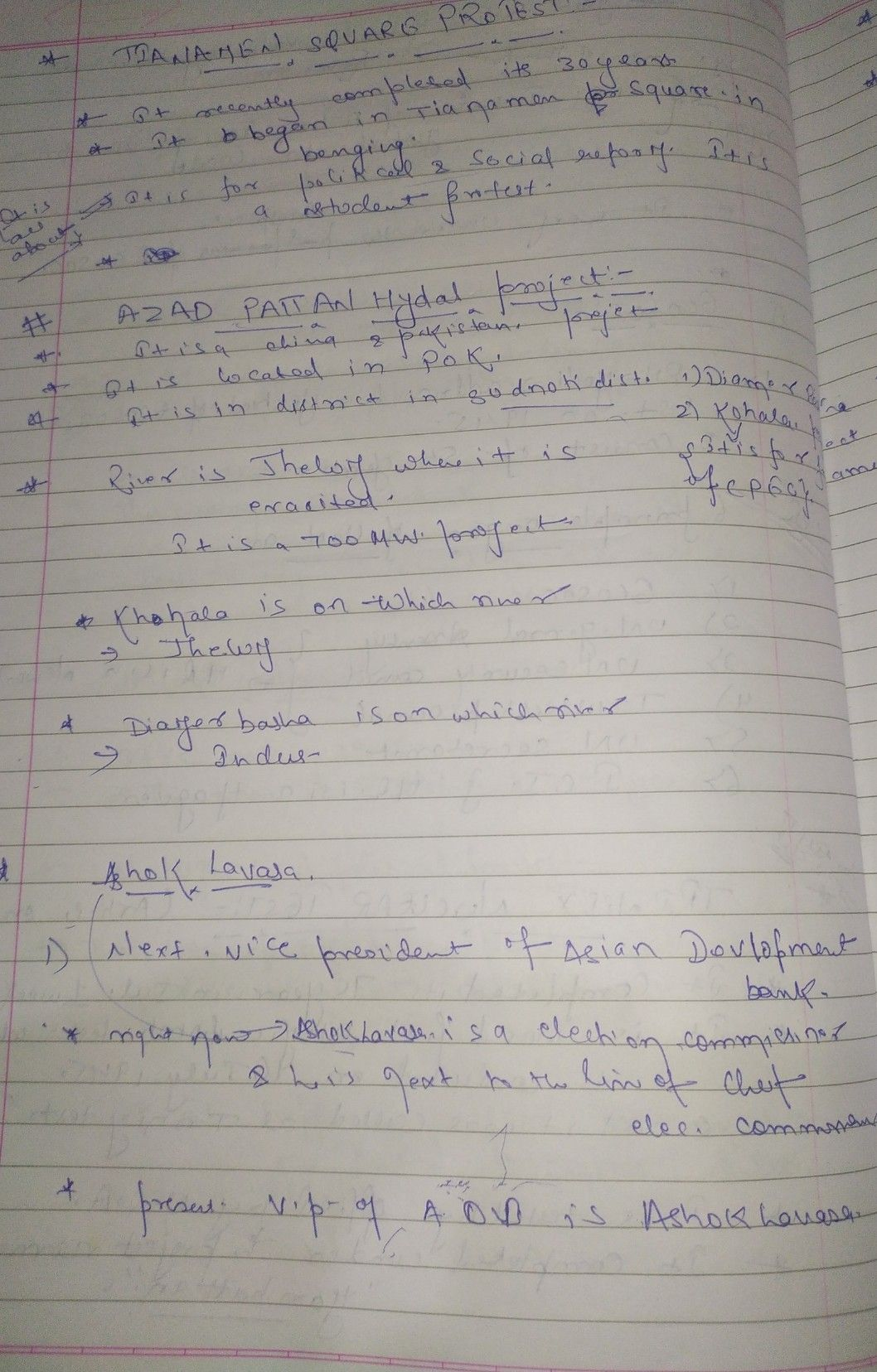Symbol
Problem$5$ $1$ Activity $5:5$ Step $oy$ Step! Work in pairs. Transform the given quadratic functions into the $f_{ormy}=a\left(x-h\right)^{2}+kby$ following the steps below. $1.$ $y=x^{2}-4x-10$ $2$ $y=3x^{2}-4x+1$ Steps $Task$ $1.$ Group the terms containing x. $2.$ Factor out $a$ $3$ Complete the expression in parenthesis to make it a perfect square trinomial. $4$ Express the perfect square trinomial $aS$ the square of a binomial $5$ Give the value of h 6. Give the value of k Did you transform the quadratic function in the $formy=a\left(x-h\right)^{2}+k3$
7th-9th grade
Other
Search count: 120
SolutionQanda teacher - Akash Aryaplease give 5 rating if you liked the answer please